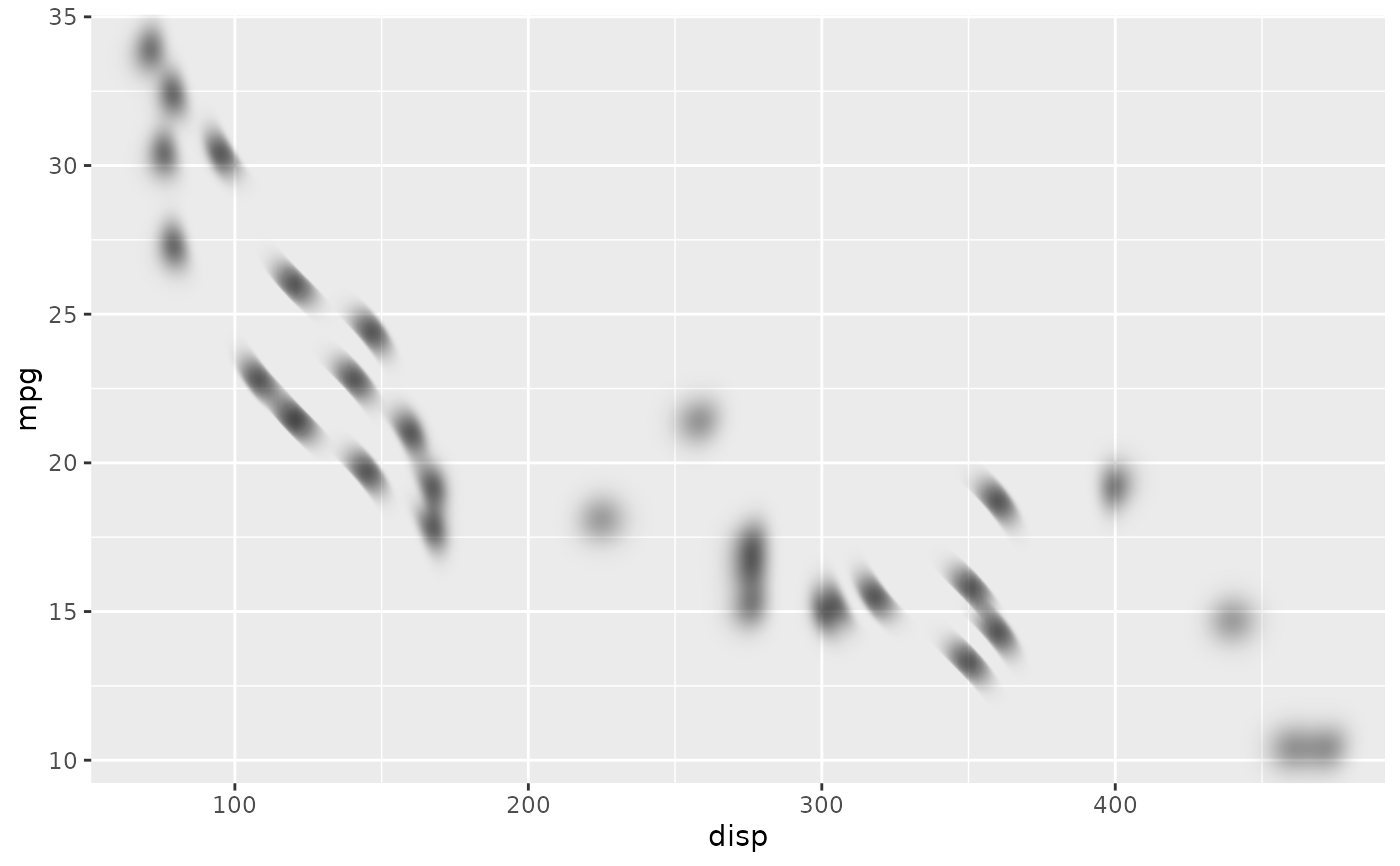This filter will blur a layer, but in contrast to with_blur() the amount and nature of the blur need not be constant across the layer. The blurring is based on a weighted ellipsoid, with width and height based on the values in the corresponding x_sigma and y_sigma layers. The angle of the ellipsoid can also be controlled and further varied based on another layer.

with_variable_blur(
x,
x_sigma,
y_sigma = x_sigma,
angle = NULL,
x_scale = 1,
y_scale = x_scale,
angle_range = 0,
...
)

## Arguments

x

A ggplot2 layer object, a ggplot, a grob, or a character string naming a filter

x_sigma, y_sigma, angle

The layers to use for looking up the sigma values and angledefining the blur ellipse at every point. Can either be a string identifying a registered filter, or a raster object. The maps will be resized to match the dimensions of x. Only one channel will be used - see the docs on channels for info on how to set them.

x_scale, y_scale

Which sigma should a maximal channel value correspond to? If a numeric it will be interpreted as pixel dimensions. If a unit object it will be converted to pixel dimension when rendered.

angle_range

The minimum and maximum angle that min and max in the angle layer should correspond to. If angle == NULL or only a single value is provided to angle_range the rotation will be constant across the whole layer

...

Arguments to be passed on to methods. See the documentation of supported object for a description of object specific arguments.

## Value

Depending on the input, either a grob, Layer, list of Layers, guide, or element object. Assume the output can be used in the same context as the input.

Other blur filters: with_blur(), with_motion_blur()

## Examples

library(ggplot2)
cos_wave <- function(width, height) {
x <- matrix(0, ncol = width, nrow = height)
x <- cos(col(x)/100)
as.raster((x + 1) / 2)
}
ggplot() +
as_reference(
cos_wave,
id = "wave"
) +
with_variable_blur(
geom_point(aes(disp, mpg), mtcars, size = 4),
x_sigma = ch_red("wave"),
y_sigma = ch_alpha("wave"),
angle = ch_red("wave"),
x_scale = 15,
y_scale = 15,
angle_range = c(-45, 45)
)